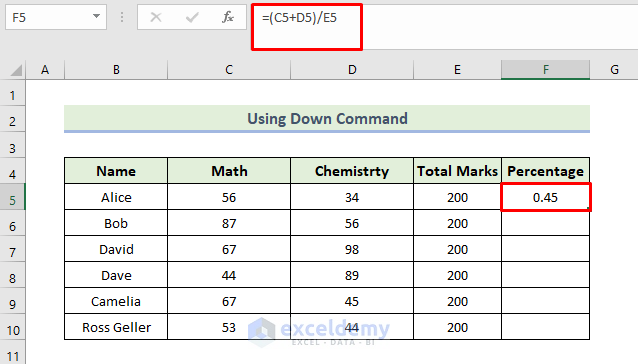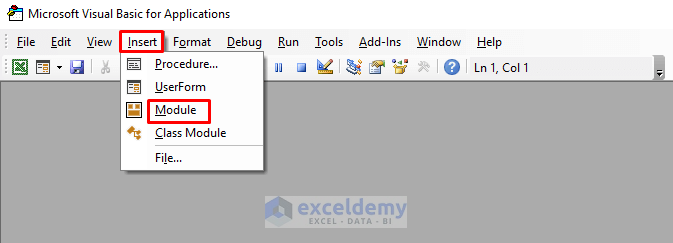# How to Fill Formula Down to Specific Row in Excel (7 Easy Methods)

Get FREE Advanced Excel Exercises with Solutions!

If you are looking for some special tricks to fill a formula down to a specific row in Excel, you’ve come to the right place. There are numerous ways to fill formulas down to particular rows in Microsoft Excel. This article will discuss six methods to fill formulas down to specific rows in Excel. Let’s follow the complete guide to learn all of this.

## 7 Suitable Methods to Fill Formula Down to Specific Row in Excel

We will use seven effective and tricky methods to fill formulas down to specific rows in Excel. This section provides extensive details on six methods. You should learn and apply all of these, as they improve your thinking capability and Excel knowledge

### 1. Dragging Fill Handle

It is the quickest and simplest way to fill formulas down to specific rows in Excel. Here, we have a dataset containing different student marks in Chemistry and Math courses and total marks. At first, we would like to calculate the total marks in terms of percentage for the first student, then fill formulas on specific rows so that each student’s percentage is calculated. We must follow the following process if we wish to fill formulas down to specific rows in Excel in the Percentage column.📌 Steps:

• Firstly, we will use the following formula in the cell F5:

`=(C5+D5)/E5`

Here, cells C5, and D5 show the marks of each student. The Total Marks are shown in cell E5.

• Press Enter.• Next, go to the Home tab, and select the Percentage symbol under the Number ribbon to convert the format of the number in cell F5 to percentage format.• Consequently, we can convert the data into percentage terms.• After that, drag the Fill Handle icon to use Excel’s AutoFill feature.• Finally, you will be able to fill formulas down to specific rows and get the percentage values in the corresponding cells.Read More: [Solved]: Fill Handle Not Working in Excel (5 Simple Solutions)

### 2. Double-Clicking on Fill Handle

Here, we will use another method to fill formulas down to specific rows in Excel. Initially, we will calculate the total marks in terms of percentage for the first student in the following dataset, then fill formulas on specific rows so that each student’s percentages can be calculated. Let’s walk through the steps to fill formulas down to specific rows in Excel.

📌 Steps:

• Firstly, we will use the following formula in the cell F5:

`=(C5+D5)/E5`

Here, cells C5, and D5 show the marks of each student. The Total Marks are shown in cell E5.

• Press Enter.• Next, go to the Home tab, and select the Percentage symbol under the Number ribbon to convert the format of the number in cell F5 to percentage format.• Consequently, we can convert the data into percentage terms.
• After that, double-click the Fill Handle icon to use Excel’s AutoFill feature.• As a result, you will be able to fill formulas down to specific rows and get the percentage values in the corresponding cells.Read More: How to Use Fill Handle to Copy Formula in Excel (2 Useful Examples)

### 3. Using Down Command from the Fill Drop-Down

In this method, we will use the Down Command from the Editing group to fill the formula down to the specific rows. Let’s walk through the steps to fill formulas down to specific rows in Excel.

📌 Steps:

• Firstly, we will use the following formula in the cell F5:

`=(C5+D5)/E5`

Here, cells C5, and D5 show the marks of each student. The Total Marks are shown in cell E5.

• Press Enter.

####• Next, go to the Home tab, and select the Percentage symbol under the Number ribbon to convert the format of the number in cell F5 to percentage format.• Consequently, we can convert the data into percentage terms.
• After that, select the range of the cells where we want to fill the formula. Then, go to the Home tab and select Editing>Fill>Down Arrow.• As a result, you will be able to fill formulas down to specific rows and get the percentage values in the corresponding cells.Read More: [Fixed!] Excel VLOOKUP Drag Down Not Working (11 Possible Solutions)

### 4. Utilizing Fill Series Command

In this method, we will use the Series command from the Editing group to fill the formula down to the specific rows. Let’s walk through the steps to fill formulas down to specific rows in Excel.

📌 Steps:

• Firstly, we will use the following formula in the cell F5:

`=(C5+D5)/E5`

Here, cells C5, and D5 show the marks of each student. The Total Marks are shown in cell E5.

• Press Enter.• Next, go to the Home tab, and select the Percentage symbol under the Number ribbon to convert the format of the number in cell F5 to percentage format.• Consequently, we can convert the data into percentage terms.
• After that, select the range of the cells where we want to fill the formula. Then, go to the Home tab and select Editing > Fill > Series.• When the Series dialog box appears, select Columns in the Series in section and select AutoFill in the Type section.• Finally, you will be able to fill formulas down to specific rows and get the percentage values in the corresponding cells.### 5. Applying Name Box

Here, we will use another method to fill formulas down to specific rows in Excel by using the Name Box option.

Let’s walk through the steps to fill formulas down to specific rows in Excel.

📌 Steps:

• Firstly, we will use the following formula in the cell F5:

`=(C5+D5)/E5`

Here, cells C5, and D5 show the marks of each student. The Total Marks are shown in cell E5.

• Press Enter.• Next, go to the Home tab, and select the Percentage symbol under the Number ribbon to convert the format of the number in cell F5 to percentage format.• Consequently, we can convert the data into percentage terms.
• Next, press ‘Ctrl+C’ to copy the cell.
• After that, in the Name Box enter the range of the cells F5:F10 where we want to fill the formula.
• Press Enter.• As a consequence, the following F5:F10 cells will be selected.• Then, press ‘Ctrl+V’.
• As a result, you will be able to fill formulas down to specific rows and get the percentage values in the corresponding cells.Read More: How to Drag Formula and Ignore Hidden Cells in Excel (2 Examples)

### 6. Using Keyboard Shortcut

Here, we will use another method to fill formulas down to specific rows in Excel by using the Keyboard Shortcut. It is the quickest and fastest way to do the task. Let’s walk through the steps to fill formulas down to specific rows in Excel.

📌 Steps:

• Firstly, we will use the following formula in the cell F5:

`=(C5+D5)/E5`

Here, cells C5, and D5 show the marks of each student. The Total Marks are shown in cell E5.

• Press Enter.• Next, go to the Home tab, and select the Percentage symbol under the Number ribbon to convert the format of the number in cell F5 to percentage format.• Consequently, we can convert the data into percentage terms.
• After that, Press ‘Ctrl+D’ to use Excel’s AutoFill feature.• As a result, you will be able to fill formulas down to specific rows and get the percentage values in the corresponding cells.Read More: How to Drag Formula in Excel with Keyboard (7 Easy Methods)

### 7. Embedding VBA Code

By using a simple code, you will be able to fill the formula down to a specific row in Excel. You have to follow the following steps.

📌 Steps:

• Firstly, press Alt+F11 to open the VBA editor. Select Insert > Module.

###• Next, you have to type the following code
``````Sub Fill_Handle()
Range("F5:F10").Formula = "=(C5+D5)/E5"
End Sub``````
• Afterward, close the Visual Basic window.
• After that press Alt+F8.
• When the Macro dialogue box opens, select Fill_Handle in the Macro name. Click on Run.• As a result, you will be able to fill formulas down to specific rows and get the percentage values in the corresponding cells.## Conclusion

That’s the end of today’s session. I strongly believe that from now you may fill the formula down to a specific row in Excel. If you have any queries or recommendations, please share them in the comments section below.

Don’t forget to check our website Exceldemy.com for various Excel-related problems and solutions. Keep learning new methods and keep growing!

## Related ArticlesWelcome to my Profile. I am working on and researching Microsoft Excel right now, and I will be posting articles about it here. I received a B.Sc. in Naval Architecture and Marine Engineering from the Bangladesh University of Engineering and Technology (BUET). Having studied naval architecture, I have a strong interest in research and development. Always try to learn from different sources and come up with creative solutions.

We will be happy to hear your thoughtsAdvanced Excel Exercises with Solutions PDF